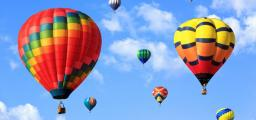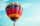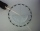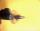# Hot air balloon

The center of the balloon is at an altitude of 600 m above the ground (AGL). From habitat on earth is the center of the balloon to see in elevation angle 38°20' and the balloon is seen from the perspective of angle 1°16'. Calculate the diameter of the balloon.

D =  55.5071 m

### Step-by-step explanation:Did you find an error or inaccuracy? Feel free to write us. Thank you!Tips to related online calculators

#### You need to know the following knowledge to solve this word math problem:

We encourage you to watch this tutorial video on this math problem:

## Related math problems and questions:

• Elevation anglesFrom the endpoints of the base 240 m long and inclined at an angle of 18° 15 ', the top of the mountain can be seen at elevation angles of 43° and 51°. How high is the mountain?
• Balloon and bridgeFrom the balloon, which is 92 m above the bridge, one end of the bridge is seen at a depth angle of 37° and the second end at depth angle 30° 30 '. Calculate the length of the bridge.
• Altitude angleIn complete winds-free weather, the balloon took off and remained standing exactly above the place from which it took off. It is 250 meters away from us. How high did the balloon fly when we see it at an altitude angle of 25°?
• AircraftThe plane flies at altitude 6500 m. At the time of first measurement was to see the elevation angle of 21° and second measurement of the elevation angle of 46°. Calculate the distance the plane flew between the two measurements.
• Two forcesTwo forces with magnitudes of 25 and 30 pounds act on an object at 10° and 100° angles. Find the direction and magnitude of the resultant force. Round to two decimal places in all intermediate steps and your final answer.
• AircraftFrom the aircraft flying at an altitude of 500m, they observed places A and B (located at the same altitude) in the direction of flight at depth angles alpha = 48° and beta = 35°. What is the distance between places A and B?
• CloudsApproximately at what height is the cloud we see under an angle of 26°10' and see the Sun at an angle of 29°15' and the shade of the cloud is 92 meters away from us?
• FighterA military fighter flies at an altitude of 10 km. The ground position was aimed at an altitude angle of 23° and 12 seconds later at an altitude angle of 27°. Calculate the speed of the fighter in km/h.
• CloudsFrom two points A and B on the horizontal plane was observed forehead cloud above the two points under elevation angle 73°20' and 64°40'. Points A , B are separated by 2830 m. How high is the cloud?
• Refractive indexThe light passes through the interface between air and glass with a refractive index of 1.5. Find: (a) the angle of refraction if light strikes the interface from the air at an angle of 40°. (b) the angle of refraction when light strikes the glass interfa
• Angles of elevationFrom points A and B on level ground, the angles of elevation of the top of a building are 25° and 37° respectively. If |AB| = 57m, calculate, to the nearest meter, the distances of the top of the building from A and B if they are both on the same side of
• ParatrooperAfter the parachute is opened, the paratrooper drops to the ground at a constant speed of 2 m/s, with the sidewinding at a steady speed of 1.5 m/s. Find: a) the magnitude of its resulting velocity concerning the ground, b) the distance of his land from a
• BallThe soldier fired the Ball at an angle of 35° at an initial velocity of 292 m/s. Determine the length of the litter. (g = 9.81 m/s2).
• Altitude anglesCities A, B, C lie in one elevation plane. C is 50 km east of B, B is north of A. C is deviated by 50° from A. The plane flies around places A, B, C at the same altitude. When the aircraft is flying around B, its altitude angle to A is 12°. Find the altit
• MoonWe see Moon in the perspective angle 28'. Moon's radius is 1740 km at the time of the full moon. Calculate the mean distance of the Moon from the Earth.
• The pondWe can see the pond at an angle 65°37'. Its end points are 155 m and 177 m away from the observer. What is the width of the pond?
• Resultant forceCalculate mathematically and graphically the resultant of three forces with a common center if: F1 = 50 kN α1 = 30° F2 = 40 kN α2 = 45° F3 = 40 kN α3 = 25°